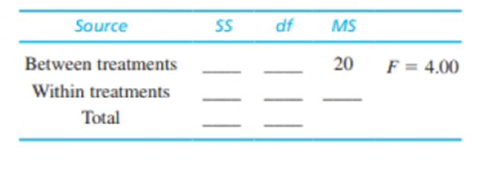Chapter 12, Problem 17PEssentials of Statistics for the B...

8th Edition
Frederick J Gravetter + 1 other
ISBN: 9781133956570

Solutions

Chapter
SectionEssentials of Statistics for the B...

8th Edition
Frederick J Gravetter + 1 other
ISBN: 9781133956570
Textbook Problem

A pharmaceutical company has developed a drug that is expected to reduce hunger. To test the drug, two samples of rats are selected with n = 20 in each sample. The rats in the first sample receive the drug every day and those in the second sample are given a placebo. The dependent variable is the amount of food eaten by each rat over a 1-month period. An ANOVA is used to evaluate the difference between the two sample means and the results are reported in the following summary table. Fill in all missing values in the table. (Hint: Start with the df column.)To determine

To find: The missing values in the ANOVA summary table given in the question.

Explanation

Given info:

Two treatment conditions with each size 20 is selected. The F-ratio is 4 and MSbetween is 20

Calculation:

Degrees of freedom for between:

dfbetween=k1=21=1

Degrees of freedom for within:

dfwithin=Nk=402=38

The value of SSbetween is calculated as follows:

MSbetween=SSbetweendfbet

Still sussing out bartleby?

Check out a sample textbook solution.

See a sample solution

The Solution to Your Study Problems

Bartleby provides explanations to thousands of textbook problems written by our experts, many with advanced degrees!

Get Started

In Exercises 13-20, sketch a set of coordinate axes and plot each point. 17. (8,72)

Applied Calculus for the Managerial, Life, and Social Sciences: A Brief Approach

Evaluate the indefinite integral. a+bx23ax+bx3dx

Single Variable Calculus: Early Transcendentals

True or False: The slope of a tangent line may be interpreted as average velocity.

Study Guide for Stewart's Single Variable Calculus: Early Transcendentals, 8th